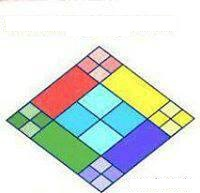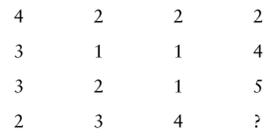Online Play Puzzles
Online Play Sudoku
Numbering Puzzles
Missing Letters Puzzles
Logical Puzzles
Placement Paper
Company Placement Paper
Gate Paper
Miscellneous
Best Project Ideas
Interviews Questions For Freshers
Request
Feedback

Puzzle 6:- How Many Squares?Ans:-26.
Sol:-Count Carefully.

Puzzle 7:- What number should replace the question mark?Ans:-9.
Sol:-The number at the centre of each triangle equals the sum of the lower two numbers minus the top number.

Puzzle 8:- What number should replace the question mark?Ans:-3.

Sol:-A+B=76 and A-B=38 then
A=57 And B=19
A/B=57/19=3

Puzzle 9:- What number should replace the question mark?Ans:-1.

Sol:-Multiply the bottom two numbers then take the square root, i.e.
1×4 = 4 and √4 = 2.

Puzzle 10:- What number should replace the question mark?Ans:-10.

Sol:-Each diagonal line of numbers, starting with the top left hand corner number, increases by 1 each time.
Example
4
2+3=5
2+1+3=6
2+1+2+2=7
4+1+3=8
5+4=9
10